﻿ Effect of Interface Morphology on Charge Transport

### Effect of Interface Morphology on Charge Transport

O.P. Garg, Vijay Kr Lamba, D.K. Kaushik

## Effect of Interface Morphology on Charge Transport

O.P. Garg1, Vijay Kr Lamba2,, D.K. Kaushik3

1RKSD College Kaithal

2Global College of Engineering & Technology, Khanpur Khui, Punjab

### Abstract

Molecular devices are a promising candidate for new technology nowadays. Great effort has been devoted recently to understand the charge transport at the interfaces in nano junctions and the role of bond length on the transport properties of molecular junctions. However, these studies have been largely based on the analysis of the low-bias conductance, which does not allow elucidating the exact influence of the symmetry in both the electronic structure and transport characteristics of the interfaces. In this work we had presented a theoretical study of the charge transport, and how conductance changes with varying the bond length of end group anchor (thiol group) on both sides of anthracene molecule to the extreme limits beyond these either the bond broke or it got overlapped with another consecutive bond. Further we rotated (along Z axis) the molecule under observation (anthracene di-thiol) and measured the effect of the rotation on the current and conductance. We have performed first principles calculations of the transport properties of these molecules using a combination of density functional theory and non-equilibrium Green's function techniques.

• O.P. Garg, Vijay Kr Lamba, D.K. Kaushik. Effect of Interface Morphology on Charge Transport. International Journal of Physics. Vol. 3, No. 4, 2015, pp 170-174. http://pubs.sciepub.com/ijp/3/4/6
• Garg, O.P., Vijay Kr Lamba, and D.K. Kaushik. "Effect of Interface Morphology on Charge Transport." International Journal of Physics 3.4 (2015): 170-174.
• Garg, O. , Lamba, V. K. , & Kaushik, D. (2015). Effect of Interface Morphology on Charge Transport. International Journal of Physics, 3(4), 170-174.
• Garg, O.P., Vijay Kr Lamba, and D.K. Kaushik. "Effect of Interface Morphology on Charge Transport." International Journal of Physics 3, no. 4 (2015): 170-174.

 Import into BibTeX Import into EndNote Import into RefMan Import into RefWorks

12
Prev Next

### 1. Introduction

With the decrease in the sizes, the electronic devices present tantalising perspectives, however the road ahead might be quite bumpy. Firstly, new fabrication methods need to be devised as we are reaching the limits in size resolution for current ones. Secondly, when modelling atomic scale circuits, one must introduce a treatment in terms of wave functions and transmission probabilities, an aspect usually ignored in conventional electronic engineering. The modelling of charge transport in systems in which one or more of the relevant length scales are of the order of a nanometer is a very challenging task. To see why this is the case, let's consider a system in which a molecule is in contact with two macroscopic metal electrodes and a battery is attached to the electrodes in order to drive a current through the system. Here we expect the charge transport in such a device to depend on the properties of the molecule, like its geometry, the character of the bonds in the molecule, the geometric details of the metal/molecule interface and the charge transfer between metal and molecule. Considering a two-probe device [1, 2, 3] (Figure 1) consisting of two charge reservoirs bridged by a nanoscale i.e. a molecule or group of molecules. Considering, the three different perspectives, (i.e. thermodynamical, quantum mechanical and electrostactical views).Download asVeiw figureFigures index
Figure 1. Schematic representation of the charge transport models under thermo-dynamical and electrostactical perspectives

From a thermodynamic point of view the system comprises two bulk leads and a central region. The central cluster, which is known as an extended molecule (EM), consists of a molecular wire in addition to few layers of surface atoms of two electrodes. We used such arrangement to:

•  include the effects of molecule & metal in self-consistent calculations,

•  include “extended molecule” assumption to correct the drop of voltage through the molecule, and

•  simplify the complexity & reduce the calculation time by using the concept of extended molecule.

The two current/voltage leads are kept at two different chemical potentials respectively μL and μR and are able to exchange electrons with the EM. Note that when the applied bias is zero, i.e. μL = μR, this system of interacting electrons is in thermodynamic equilibrium and may be regarded as a grand canonical ensemble. When the bias is applied however μLμR and the current will flow. The prescription for establishing the steady state is that of adiabatically switching on the coupling between the leads and the EM [2, 3, 4, 5]. Let a battery be connected to the ends of the charge reservoirs and a potential difference is applied. Effectively this bias is what keeps the two chemical potentials μL and μR different. The effect will be of charge flowing across the device, from one reservoir into another to try to counter-balance the effect of the current.

Therefore, in order to achieve a good match of the electrostatic potential, several layers of the electrode are included in the extended molecule. Their number ultimately depends upon the screening length of the electrode, but in most situations we take between two and four atomic planes. In order to find the electron’s wavefunction and determine the full quantum mechanical properties of the problem one, in principle, needs to diagonalise H. We used Green function [6, 7, 8, 9] to calculate the electronic properties of an open system. In general, the electron density matrix in real space is given by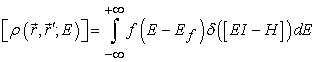(1)

Here δ(EI-H) is the local density of states. We write the standard expansion for the delta function as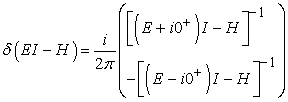(2)

Equation (2) can also be written in the form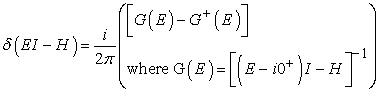(3)

G(E) is the retarded Green’s function while G+(E), its conjugate complex transpose. The diagonal elements of the spectral function, which is the difference between the retarded and advanced Green’s function, represent the available local electron density of states.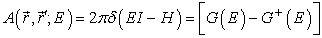(4)

The electron density in the channel is the product of the Fermi function and the available density of states and for an isolated device is written as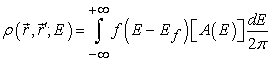(5)

To understand the process of current flow, consider an isolated device having a single energy level ε. The source and drain contacts have an infinite distribution of electronic energy states. When the isolated device with single energy level ε is connected to the source and drain contacts, some of the density of states around this energy level ε will spill over from the contacts into the channel. This process is known as energy level broadening. If the Fermi levels in the source and drain are equal, the amount of broadening will be equal on both sides and hence the net current flow in the channel will be zero. When a positive bias is applied on the drain side, the Fermi level on the drain side is lowered according to equation (5), opening up states below the channel energy level ε in the drain.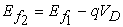(6)

The electrons entering the channel with energy ε now have states with lower energies available in the drain to escape to. This causes the channel current to become non-zero. As the applied bias is increased linearly, more and more states between ε and εf2 become available to remove electrons from the channel causing the source to increase its supply of electrons into the channel. This phenomenon results in a linear increase of current in the channel. The current reaches saturation such that the number of electrons leaving the drain will equal the number of electrons entering from the source. This process also explains why experimental measurements [10, 11, 12, 13] have shown that the maximum measured conductance of a one-energy level channel approaches a limiting value G0= 2q2/ ћ = 51.6(KΩ)-1. Since the coupling of the device to the source and drain contacts is described using self-energy matrices Σ1, Σ2. The self-energy term can be viewed as a modification to the Hamiltonian to incorporate the boundary conditions. Accordingly, equation [14, 15, 16, 17] (2) and (6) can be rewritten as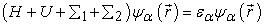(7)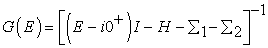(8)

The self-energy terms Σ1 and Σ2 originate from the solution of the contact Hamiltonian. The broadening of the energy levels introduced by connecting the device to the source and drain contacts is incorporated through the Gamma functions Γ1 and Γ2 given by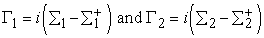(9)

The electron density for the open system is now given by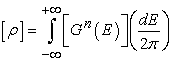(10)

Gn(E) represents the electron density per unit energy and is given by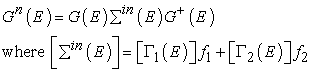(11)

The current through the channel is calculated as the difference between the inflow and the outflow at any given contact.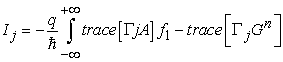(12)

where the subscript j indexes the contacts. For a two-terminal device I1 = −I2.

Even if one is able to calculate the Hamiltonian H the problem of transport still needs to be addressed. We do so by looking at two different limits.

Firstly the limit of infinitesimally small bias: the linear regime. Here, we can formulate a quantum mechanical description of a system lying at or close to equilibrium conditions. We assume that under an external applied bias the Hamiltonian H(V) does not significantly change from that of the ground-state H(V = 0). Here we use Landauer-Buttiker formalism [14, 15, 16, 17] to calculate the transport properties which is given by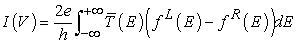(13)

where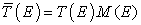.

Secondly, we can use a very simple model of transport across a single energy level in order to understand changes to the system’s Hamiltonian under bias. The total current at steady state can be associated with the rate of transfer of electrons across the EM. These two simple cases will lead to a deeper understanding of the transport problem. We will then join these two concepts into a more general framework and design the model.Download asVeiw figureFigures index
Figure 2. Molecular junction made of an anthracene molecule stringed between two gold electrodes with thiol linker group. Here we used 3X3 gold electrode with crystal orientation of [1,1,1]

We have considered an anthracene molecular wire with thiol end groups at para position bonded to two gold electrodes as shown in Figure 2. The choice of the thiol anchoring groups ensures a strong chemical bonding to the leads with the current flow mainly modulated by the molecular HOMO-LUMO level [18, 19, 20]. We had applied a bias voltage in a range of -2 V to 2 V across the molecular junction.

### 2. Computational Method

Our junction consists of anthracene molecule stringed to gold electrodes through the thiol linkers. We varied three vital aspects of geometry and observed the:

1. Effect of varying the bond length of endgroup anchor (thiol group) on both sides of anthracene molecule to the extreme limits beyond these either the bond broke or it got overlapped with another consecutive bond;

2. We varied the bonding angle between molecule under observation (anthracene di-thiol) and the gold electrodes and measured the effect of these changes on the current and conductance, and;

3. The effect of changing rotation angle between the molecules under observation.

After the initial geometry optimization as the force and stress on the molecule under observation approached 0.1 eV/A0, the transport metrics of current and conductance were measured separately under the variegated applied bias.

The bond length of linker atom sulphur attached to carbon atom at either end of anthracene molecule was varied and we observed the two extreme limits of this bond to exist. The length was varied from 0.88 A0 on the lower end to 2.14 A0on the higher end. These limits were computed from the default bond length of corresponding carbon and sulphur atoms i.e. 1.098 A0, once by elongating the bond length such that the bond reached the limits to break and then by decreasing it to an extent until it got overlapped by consecutive bonds. The corresponding values of current and conductance were calculated using equation (12 & 13) and shown in Table 1 below.

#### Table 1. Values of conductance & current with corresponding Bond lengths (A°)Download asVeiw tableTables index

### 3. Results & Discussions

We observed that the conductance showed least values of 7.32 µS and 5.212 µS at the extreme limits of bond length i.e. 0.88 A0 and 2.14 A0 respectively whereas the maximum conductance was observed for the C-S bond length of 1.24 A0. Similarly the current values were observed to be decreasing as we increase molecule/electrode distance. From the Figure 3, and Table 1, we observed that he maximum value of conductance was reported as 70.75 µS approx. 0.9 G0 (quantum conductance) at bond length 1.24 A0. The observed value of conductance at this bond length meant that the coupling between the molecule and the metallic electrodes is tightest for this bond length though it still remained the loose coupling and contacts showed a tendency to become metallic from tunnel contacts. Further explanation confirmed that there was linear decrease in conductance when the bond length was decremented from 1.24 A0 towards the extremity 0.88A0. As the bond length was incremented from 1.24A0 towards the other extremity having highest value, it again showed a linear decrease initially and exhibited a falling slope towards highest limit of bond length.

The reduced conductance was recorded for bond length 2.14 A0 when the bond was elongated maximum. This observed conductance along with very fragile current indicated weakest coupling among the molecule and the electrodes. Hence we concluded here that the bond length of the end group linker with the molecule under consideration played a vital role for the optimal nanometer-scale electron transport through this molecular junction.

By varying the bond angle between anthracene molecule and the semi-infinite gold electrodes in discrete steps from 0 to 50 degrees, leads to the distorting of the electronic structure of the system under equilibrium. The corresponding values of conductance and current have been shown in Figure 4. Since the conduction in anthracene is due to π-conjugated molecular orbital’s, which extend over the whole molecule and facilitate the transport of electrons between the two electrodes. As they are made mostly of anisotropic p atomic orbital, the overlap of the conducting MO’s with electrode is a function of the angle between the main axis of the molecule and the normal surface. In general, we expect the overlap and the full conductance to be maximal when the lobes of the p orbital of the end atom at the molecule are oriented perpendicular to the surface and smaller otherwise, as dictated by the symmetry. The conductance is proportional to the square of the matrix element, which contains a product of two metal-molecule hopping integrals; the total conductance variation with overall geometry may therefore reach two orders of magnitude, and in special cases be even larger, as shown in Figure 4.Download asVeiw figureFigures index
Figure 3. Effect of Variation of distance between Electrode surface and molecule on transport propertiesDownload asVeiw figureFigures index
Figure 4. Effect of tilt angle on charge transport properties of molecular junction

The conclusion would be only valid when the electronic structure of the molecule and the molecule-electrode distance does not change significantly while its orientation is being changed. In this work the molecular wire is rotated about the bottom ‘‘atom’’ and thereby changing the angle θ between the main axis of the chain and the surface normal to it. As θ changes, the p orbital remains oriented perpendicular to the molecular backbone. The molecule-electrode bond lengths do not change with θ, and the surfaces of the two electrodes always remain parallel, and the gap between LUMO and HOMO is ELUMO EHOMO = 2tπ (were tπ is hopping between those π orbitals). The middle of the gap is aligned with the chemical potential of the probe at zero bias voltage (V = 0). From the Figure 3, we conclude that antharacene with planar structures have much better conductance than the molecule with twist structures due to smaller band gaps, better orbital orientation for electron delocalization.

Lastly, we varied the rotation angle between the anthracene and gold electrodes and scrutinized its effect on the values of current and conductance, plotted in Figure 4. We observed (Figure 5) interesting plots for the variations in rotation angle of the anthracene molecule with respect to the gold electrodes. The values of current and conductance showed fluctuations between the ranges 38.4 µA to 40.75 µA and 40.93 to 43.8 µS respectively. We all know that the aromatic organic molecules contain conjugated double bonds. The instability due to the delocalized π- electrons results in varied conjugation by subjecting rotation to the anthracene di-thiol molecule.Download asVeiw figureFigures index
Figure 5. Effect of Rotation on Conductance/Current

### 4. Conclusion

The results presented in this paper show the effects of interface morphology on charge transport across a single-molecule junction. We found that the bond length of the end group linker with the molecule under consideration played a vital role for the optimal nanometer-scale electron transport through the molecular junction. Further by varying the bond angle between anthracene molecule and gold electrodes. the conductance is proportional to the square of the matrix element, which contains a product of two metal-molecule hopping integrals. the total conductance variation with overall geometry may therefore reach two orders of magnitude, thus we conclude that antharacene with planar structures have much better conductance than the molecule with twist structures due to smaller band gaps, better orbital orientation for electron delocalization

### Acknowledgement

This work is part of the research projects supported by AICTE under Grant No. 8024/RIFD/MOD-145/2010-11 at HCTM Kaithal.

### References

  N.W. Ashcroft and N.D. Mermin, “Solid State Physics”, Saunders College Publishing, New York, 1976, Chap 2, 3.In article  C. Caroli, R. Combescot, P. Nozieres, and D. Saint-James, “A direct calculation of the tunnelling current: IV. Electron-phonon interaction effects”, J. Phys. C, 21(5), 1972.In article View Article  L. Kadanoff and G. Baym, “Quantum Statistical Mechanics”, W.A. Benjamin, Menlo Park, CA, 1962, Chapter 6-9.In article  L. V. Keldysh, “Diagram Technique for Non-equilibrium Processes” , Sov. Phys. JETP (20), P. 1018, 1965.In article  Atomistix ToolKit: Manual, 11.2.0, Chapter 4 & 5; (2011).In article  S. Datta, “Electronic Transport in Mesoscopic Systems”, Cambridge University Press, Cambridge, UK, 1995, Chapter 2, 5 & 7.In article View Article  H. Haug and A. P. Jauho, “Quantum Kinetics in Transport and Optics of Semi-Conductors”, Berlin, 1996, Chapter 8, & 9.In article  M. D. Ventra, S. T. Pantelides, and N. D. Lang, “First-Principles Calculation of Transport Properties of a Molecular Device”, Phys. Rev. Lett. (84), 2000, P 979.In article  E. Emberly and G. Kirczenow, “Theoretical study of electrical conduction through a molecule connected to metallic nanocontacts”, Phys. Rev. B (58), 1998, P 10911.In article  A.R. Rocha and S. Sanvito, “Asymmetric I-V characteristics and magnetoresistance in magnetic point contacts”, Phys. Rev. B , (70), 2004, P 220045.In article  R. Rocha, V. M. G. Su´arez, S. W. Bailey, C. J. Lambert, J. Ferrer, and S. Sanvito, “Spin and molecular electronics in atomically generated orbital landscapes”, Phys. Rev. B (73), 2006, P 085414.In article  C.W.J. Beenakker, “Theory of Coulomb-blockade oscillations in the conductance of a quantum dot”, Phys. Rev. B (44), 1991, P 1646.In article  R. Gebauer and R. Car, “ Kinetic theory of quantum transport at the nanoscale”, Phys. Rev. B (70), 2004, P 125324.In article  L. G. C. Rego, A. R. Rocha, V. Rodrigues, and D. Ugarte, “ Role of structural evolution in the quantum conductance behaviour of gold nano-wires during stretching”, Phys. Rev. B (67), 2003, P 045412.In article  M. Buttiker, Y. Imry, R. Landauer, and S. Pinhas, “Generalized many-channel conductance formula with application to small rings”, Phys. Rev. B (31), 1985, 6207.In article  B.J. Van Wees, H. Van Houten, C. W. J. Beenakker, J. G. Williamson, L. P. Kouwenhoven, D. van der Marel, and C. T. Foxon. “Quantized conductance of point contacts in a two-dimensional electron gas”, Phys. Rev. Lett. (60), 1988 P 848.In article  D. Stone and A. Szafer. “What is measured when you measure a resistance?- The Landauer formula” , IBM J. Res. Develop., (32), 1988, P-384.In article  Yun Zheng, Cristian Rivas, Roger Lake, “Electronic Properties of Silicon Nanowires”, IEEE Transactions on Electron Devices, vol. 52, no. 6, 2005, pp.1097-1103In article View Article  Sweta Parashar, Pankaj Srivastava, and Manisha Pattanaik, “Electrode materials for biphenyl-based rectification devices”, J Mol Model (19) 2013, P 4467-4475.In article  Du Y, Pan H, Wang S, Wu T, Feng YP, Pan J, Wee ATS, “Symmetrical negative differential resistance behavior of a resistive switching device”. ACS Nano 6, 2012, P 2517-2523.In article View Article  PubMed